# What is the molar solubility (in mol/L) of Cu3PO4 (Ksp = 1.56⋅10−4) in a 0.21 M CuNO3 solution. W...

What is the molar solubility (in mol/L) of Cu3PO4 (Ksp = 1.56⋅10−4) in a 0.21 M CuNO3 solution.

What is the molar solubility (in mol/L) of CuOH (Ksp = 6.32⋅10−26) in a solution buffered at 10.91.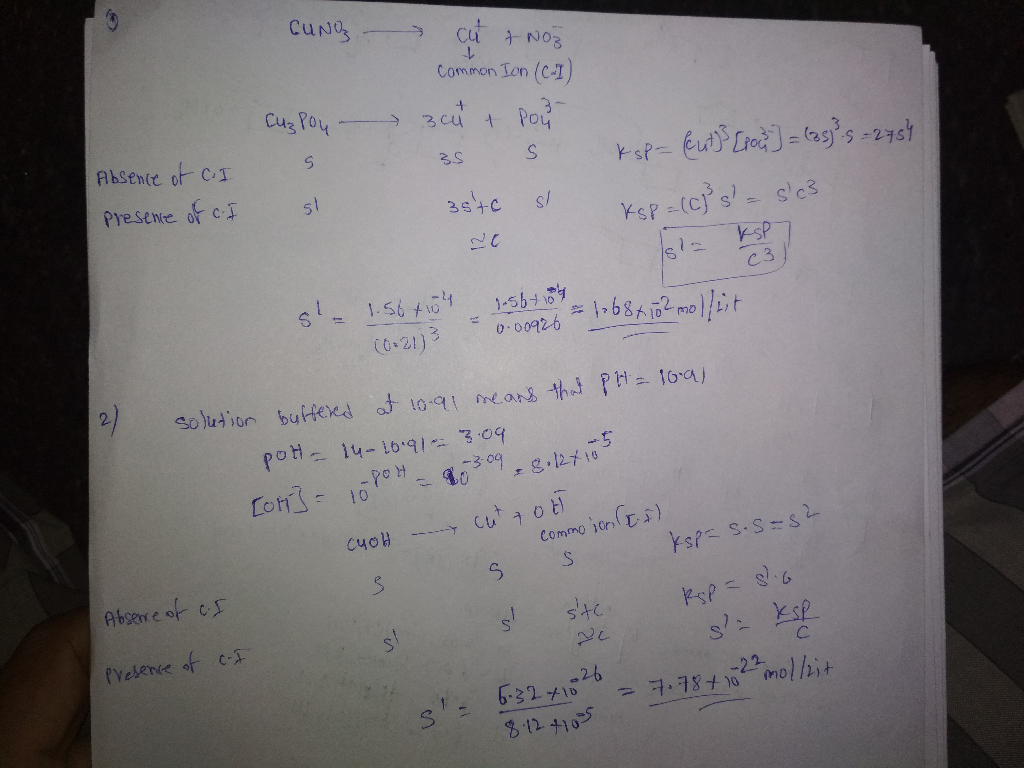##### Add Answer of: What is the molar solubility (in mol/L) of Cu3PO4 (Ksp = 1.56⋅10−4) in a 0.21 M CuNO3 solution. W...
Similar Homework Help Questions
• ### (a) If the molar solubility of ScF3 at 25 oC is 6.81e-07 mol/L, what is the...

(a) If the molar solubility of ScF3 at 25 oC is 6.81e-07 mol/L, what is the Ksp at this temperature? Ksp = (b) It is found that 0.00354 g of Ag2CO3 dissolves per 100 mL of aqueous solution at 25 oC. Calculate the solubility-product constant for Ag2CO3. Ksp = (c) The Ksp of ZnSe at 25 oC is 3.60e-26. What is the molar solubility of ZnSe? solubility =

• ### 28. -70.1 points 0/4 Submissions Used (a) If the molar solubility of YF3 at 25 °C is 4.23e-06 mol/L, what is the Ksp at...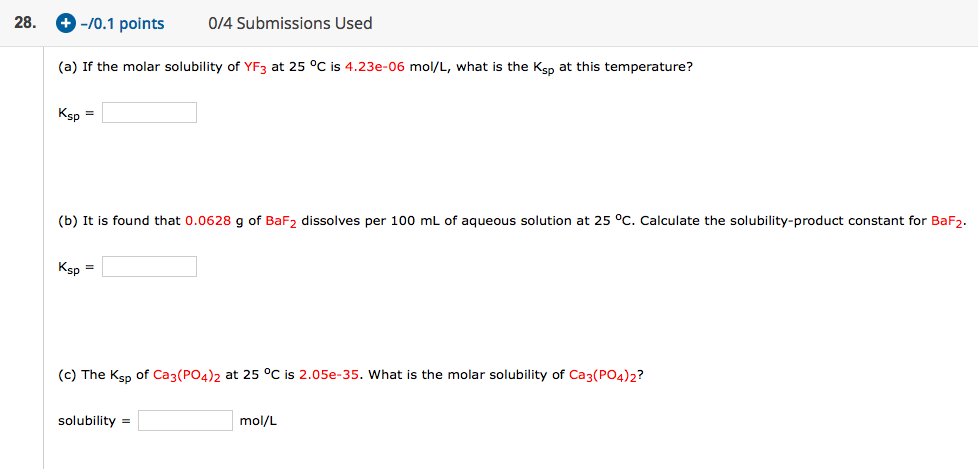28. -70.1 points 0/4 Submissions Used (a) If the molar solubility of YF3 at 25 °C is 4.23e-06 mol/L, what is the Ksp at this temperature? Ksp =D (b) It is found that 0.0628 g of BaF2 dissolves per 100 mL of aqueous solution at 25 °C. Calculate the solubility-product constant for BaF2. Ksp = (c) The Ksp of Ca3(PO4)2 at 25 °C is 2.05e-35. What is the molar solubility of Ca3(PO4)2? solubility = mol/L

• ### Calculate the molar solubility of CuSO. (Ksp = 8.1 x 10-16) a) in water b) in...Calculate the molar solubility of CuSO. (Ksp = 8.1 x 10-16) a) in water b) in 0.10 M CUNO3(aq) solution

• ### (a) If the molar solubility of BaF2 at 25 °C is 0.00358 mol/L, what is the...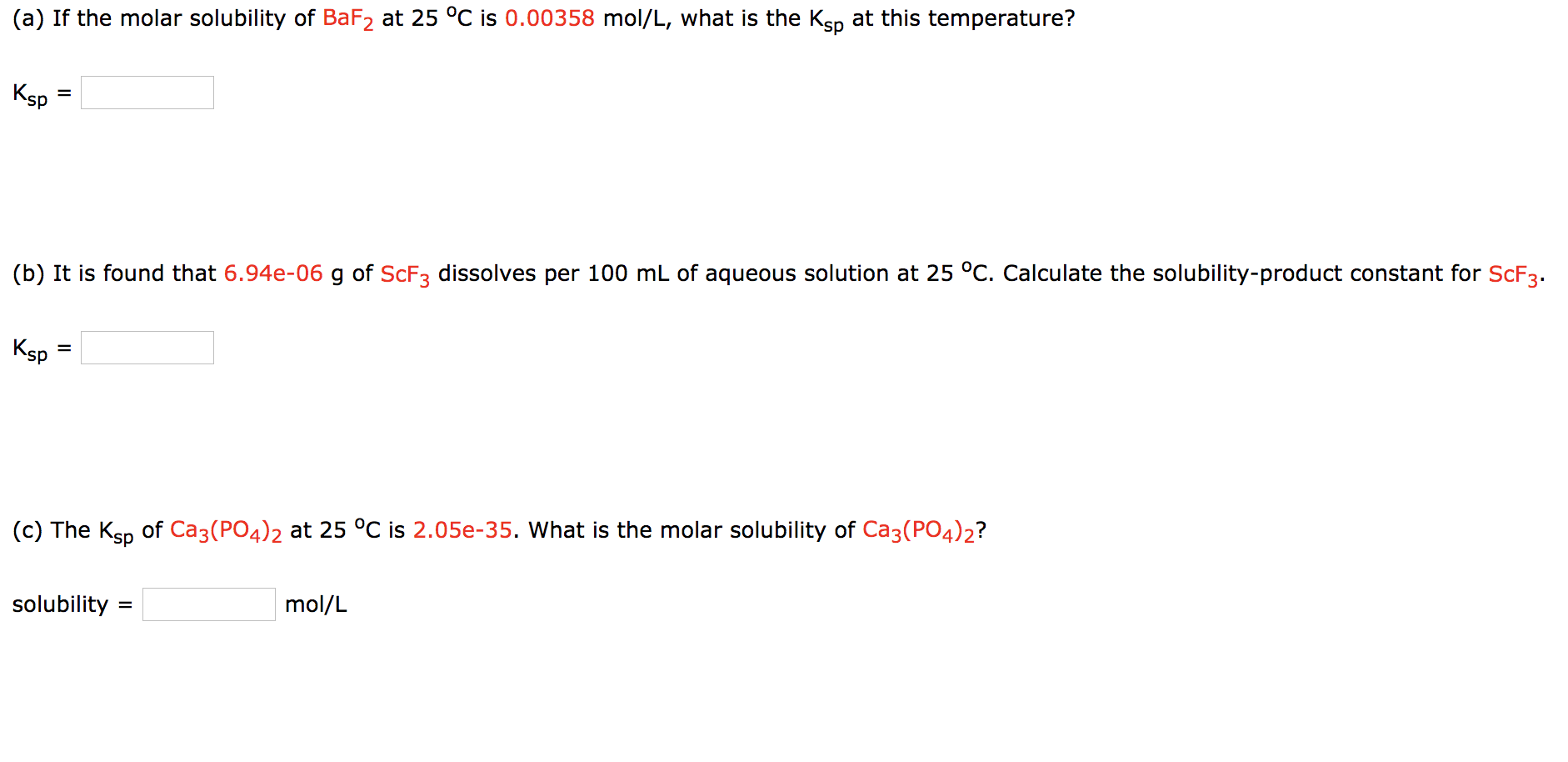(a) If the molar solubility of BaF2 at 25 °C is 0.00358 mol/L, what is the Ksp at this temperature? Ksp = (b) It is found that 6.94e-06 g of ScFz dissolves per 100 mL of aqueous solution at 25 °C. Calculate the solubility-product constant for ScF3. Ksp = (c) The Ksp of Ca3(PO4)2 at 25 °C is 2.05e-35. What is the molar solubility of Ca3(PO4)2? solubility = mol/L

• ### (a) If the molar solubility of SrC2O4 at 25 oC is 0.000224 mol/L, what is the...

(a) If the molar solubility of SrC2O4 at 25 oC is 0.000224 mol/L, what is the Ksp at this temperature? Ksp = (b) It is found that 8.70e-09 g of Ga(OH)3 dissolves per 100 mL of aqueous solution at 25 oC. Calculate the solubility-product constant for Ga(OH)3. Ksp = (c) The Ksp of ScF3 at 25 oC is 5.81e-24. What is the molar solubility of ScF3? solubility = (mol/L)

• ### (a) If the molar solubility of Tl, at 25°C is 5.31e-08 mol/L, what is the Ken at this temperature? Ksp = (b) It is foun...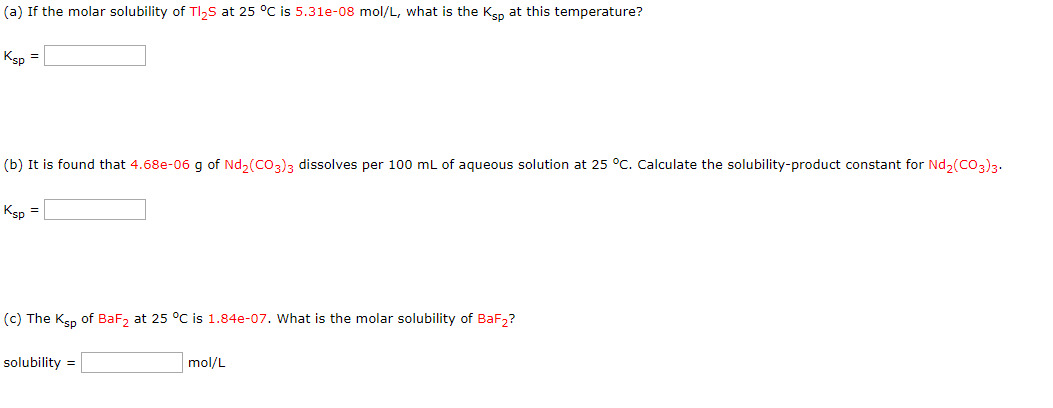(a) If the molar solubility of Tl, at 25°C is 5.31e-08 mol/L, what is the Ken at this temperature? Ksp = (b) It is found that 4.68e-06 g of Nd2(CO3)3 dissolves per 100 mL of aqueous solution at 25 °C. Calculate the solubility-product constant for Nd2(CO3)3. Ksp = (c) The Ksp of BaF2 at 25 °C is 1.84e-07. What is the molar solubility of BaFZ? solubility = mol/L

• ### Calculate the molar solubility of Cu2SO3 (Ksp = 8.1 x 10-16) a) in water b) in...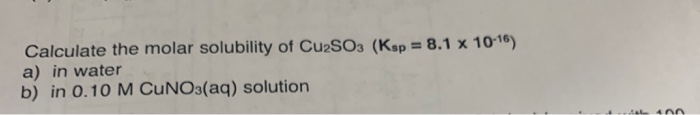Calculate the molar solubility of Cu2SO3 (Ksp = 8.1 x 10-16) a) in water b) in 0.10 M CUNO3(aq) solution IL 4A

• ### (a) If the molar solubility of PbBr2 at 25 oC is 0.0118 mol/L, what is the...

(a) If the molar solubility of PbBr2 at 25 oC is 0.0118 mol/L, what is the Ksp at this temperature? Ksp = b) It is found that 6.25e-06 g of Cd3(PO4)2 dissolves per 100 mL of aqueous solution at 25 oC. Calculate the solubility-product constant for Cd3(PO4)2. Ksp = (c) The Ksp of Sc(OH)3 at 25 oC is 2.22e-31. What is the molar solubility of Sc(OH)3? solubility = mol/L

• ### (a) If the molar solubility of Ag3AsO4 at 25 oC is 1.40e-06 mol/L, what is the...

(a) If the molar solubility of Ag3AsO4 at 25 oC is 1.40e-06 mol/L, what is the Ksp at this temperature? Ksp =   (b) It is found that 1.75e-06 g of Cu3(AsO4)2 dissolves per 100 mL of aqueous solution at 25 oC. Calculate the solubility-product constant for Cu3(AsO4)2. Ksp =   (c) The Ksp of PbBr2 at 25 oC is 6.60e-06. What is the molar solubility of PbBr2? solubility =  mol/L

• ### (a) If the molar solubility of ZnSe at 25 oC is 1.90e-13 mol/L, what is the...

(a) If the molar solubility of ZnSe at 25 oC is 1.90e-13 mol/L, what is the Ksp at this temperature? Ksp = (b) It is found that 1.77e-05 g of PbCrO4 dissolves per 100 mL of aqueous solution at 25 oC. Calculate the solubility-product constant for PbCrO4. Ksp = (c) The Ksp of Y2(CO3)3 at 25 oC is 1.03e-31. What is the molar solubility of Y2(CO3)3? solubility = mol/L

Need Online Homework Help?